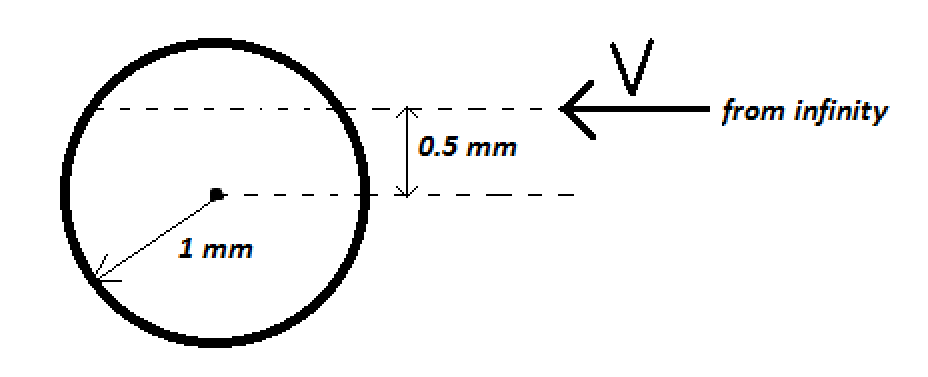# JEE Advanced Electrostatics (Non-conductors) 1A particle of mass $1\ kg$ and charge $1/3\ \mu C$ is projected towards a non-conducting fixed spherical shell having the same charge uniformly distributed on its surface. The minimum initial velocity of projection required if the particle just grazes the shell is $\psi$. Calculate $\lfloor 10^3\psi\rfloor$

×